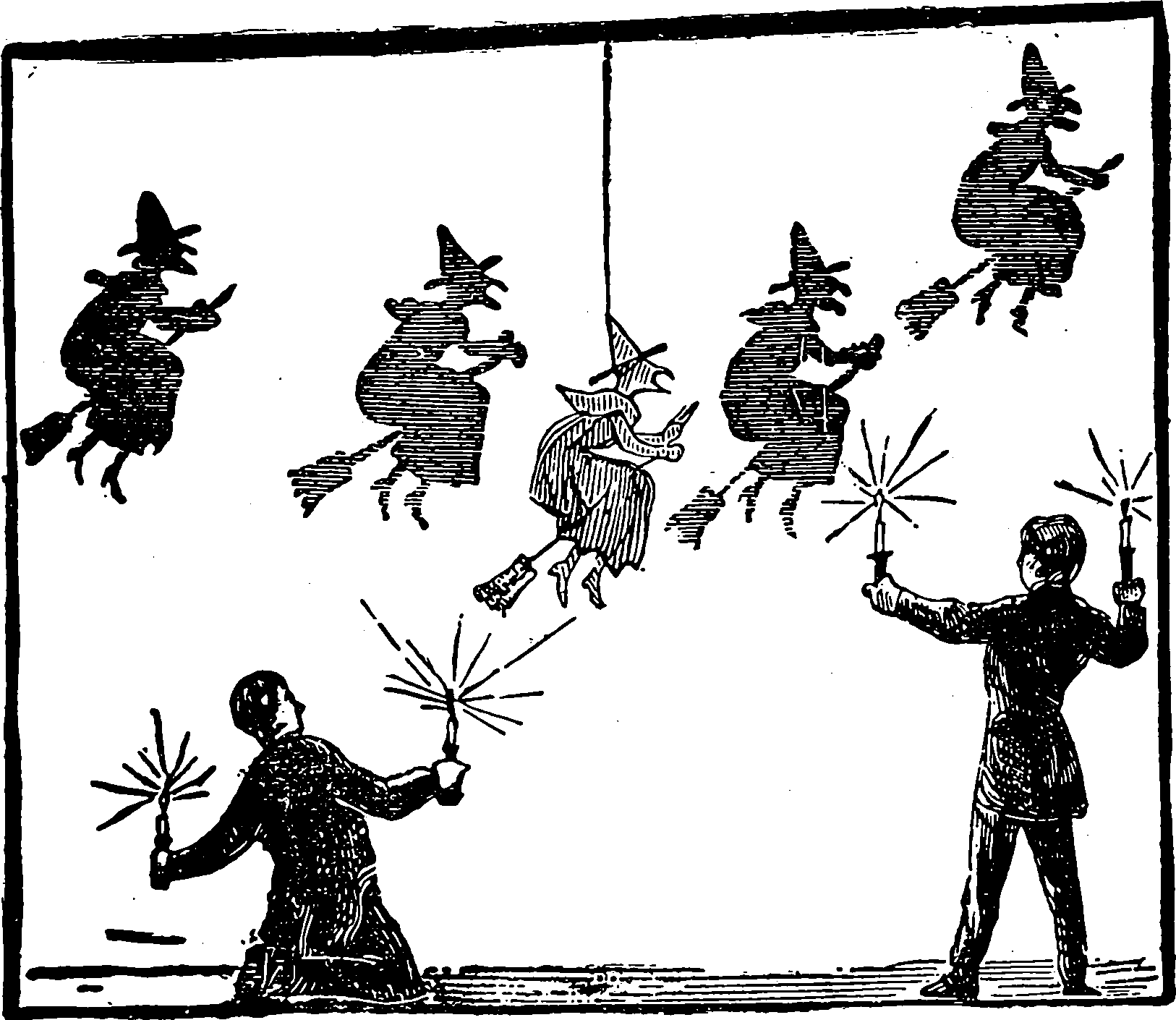# Polynomial basesPlaceholder.

You know what is a bloody great introduction to polynomial bases? Golub and Meurant (2010). They cram it into the first chapter and then do computational stuff in the subsequent chapters. It is excellent.

## Fun things

Terry Tao on Conversions between standard polynomial bases.

Representation in terms of basis is useful for analyzing the Natural exponential family with quadratic variance function .

### Well known facts

Xiu and Karniadakis (2002) mention the following “Well known facts”:

All orthogonal polynomials $$\left\{Q_{n}(x)\right\}$$ satisfy a three-term recurrence relation $-x Q_{n}(x)=A_{n} Q_{n+1}(x)-\left(A_{n}+C_{n}\right) Q_{n}(x)+C_{n} Q_{n-1}(x), \quad n \geq 1$ where $$A_{n}, C_{n} \neq 0$$ and $$C_{n} / A_{n-1}>0 .$$ Together with $$Q_{-1}(x)=0$$ and $$Q_{0}(x)=1,$$ all $$Q_{n}(x)$$ can be determined by the recurrence relation.

It is well known that continuous orthogonal polynomials satisfy the second-order differential equation $s(x) y^{\prime \prime}+\tau(x) y^{\prime}+\lambda y=0$ where $$s(x)$$ and $$\tau(x)$$ are polynomials of at most second and first degree, respectively, and $\lambda=\lambda_{n}=-n \tau^{\prime}-\frac{1}{2} n(n-1) s^{\prime \prime}$ are the eigenvalues of the differential equation; the orthogonal polynomials $$y(x)=$$ $$y_{n}(x)$$ are the eigenfunctions.

## Zoo

This list is extracted from a few places including Xiu and Karniadakis (2002).

FamilyOrthogonal wrt
Monomialn/a
Bernsteinn/a
Legendre$$\operatorname{Unif}([-1,1])$$
Hermite$$\mathcal{N}(0,1)$$
Laguerre$$x^{\alpha}\exp -x, \, x>0$$
Jacobi$$(1-x)^{\alpha }(1+x)^{\beta }$$ on $$[-1,1]$$
Gegenbauer$$\left(1-x^2\right)^{\alpha-\frac{1}{2}}$$ on $$[-1,1]$$cf Funk-Hecke formula; special case of Jacobi
Chebyshev$$\left(\sqrt{1-x^2}\right)^{\pm1}$$ on $$[-1,1]$$Special case of Gegenbauer
CharlierPoisson distribution
Meixnernegative binomial distribution
Krawtchoukbinomial distribution
Hahnhypergeometric distribution
???Unit ballDoes this have a name?

## Tools

### No comments yet. Why not leave one?

GitHub-flavored Markdown & a sane subset of HTML is supported.